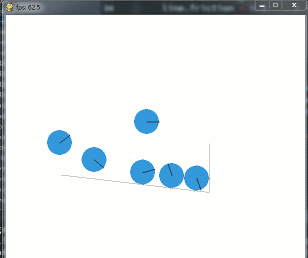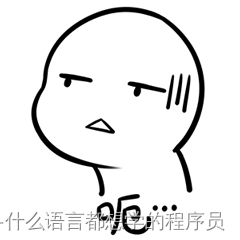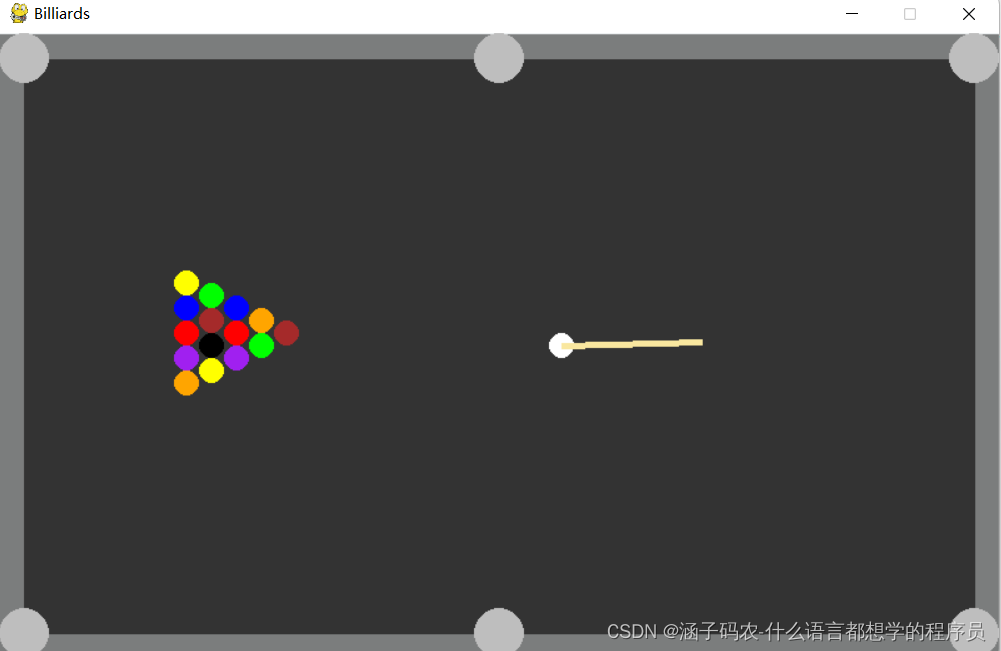### python-pygame与pymunk-台球游戏

python-编程宇宙-计算机网络的宇宙希望大家可以更加了解pymunk！

一、前面准备

三、最后程序

# 一、前面准备

``# -coding utf-8 -# import pygame, pymunk and sysimport pygame as pgimport pymunk as pmimport sys # init programpg.init()# set screenscreen = pg.display.set_mode((800, 500)) # cannot change the size of the screen# set titlepg.display.set_caption("Billiards", "4.0") # pymunk Spacespace = pm.Space()# gravity# gravity and speedspace.gravity = (0, 0) # gravity = 0 # colors for drawwhite = (236, 240, 241)  # whitegray = (123, 125, 125) # graystage = (51, 51, 51) # stage points = [""] * 16 # particleBalls balls = [""] * 16 # rigid bodies y = 0 # Ypos x = 0 # Xpos n = 5 # create n # colors, use for ballscolors = ["yellow", "blue", "red", "purple", "orange", "green", "brown", "black",          "yellow", "blue", "red", "purple", "orange", "green", "brown","white"]``

pymunk.sapce和gravity是啥？没事，我们来看看：space就是指一个空间，gravity就是重力。我们不需要重力。

# 二、中间程序

``# def drawdef draw():    # screen fill    screen.fill(white)    # draw stage    pg.draw.line(screen, gray, (0, 250), (800, 250), 500)    pg.draw.line(screen, stage, (20, 250), (780, 250), 460)    # draw a ball in the pygame, pos is the points' and balls'    # make the ball has gravity    # show the ball    # use range    for i in range(16):        # if it appears, then--        # if it doesn't, don't draw it, or system wrong         if points[i]:            # --draw circle            pg.draw.circle(screen, colors[i], points[i].position, 10)    # update space, can change ball's position    space.step(0.2) # use space.step # create holes this listholes = [(20,20),(400,20),(780,20),(20,480),(400,480),(780,480)] # def drawHoledef drawHole():    # use loop    for h in holes:        # draw circle        pg.draw.circle(screen,'gray',h,20) # def disdef dis():    # global first    global points,balls    # use loop    for i,p in enumerate(points):        # check pos        for h in holes:            # check            if p and (p.position-h)**2+(p.position-h)**2<400:                # make the ball disappear                 points[i] = ""                 balls[i] = "" # def frictiondef friction():    # make the balls speed be slower and slower    # global first!    global points    for v in points:        # if v is appear        if v:            # then make the speed slower            v.velocity *= 0.99 # def cuedef cue():    # get white ball speed(velocity)    # it is a little bit difficult    # if abs(speed) < 0.1, the do next    # because of there will have -num, so we need abs!    # first need loop!    # staticflag    staticFlag = 1    for i in points:        # check        # switch thinking is on again!        if i and (abs(i.velocity) > 0.1 or abs(i.velocity) > 0.1):            # there's a ball is running!            staticFlag = 0    # then check    if points and staticFlag == 1:        # get pos        mPos = pg.mouse.get_pos()        # draw cue        pg.draw.line(screen,(249,231,159),points.position,mPos,5)        # get if pressed        if pg.mouse.get_pressed():            # move ball and cue, and boom!            # wallop!            points.apply_impulse_at_local_point(((points.position-mPos),(points.position-mPos)),(0,0)) # create balls# def createdef create():    # global first, or system error    global points,balls    # create y, x, n    y = 0    x = 0    n = 5    # create balls of particle balls and rigid bodies    for i in range(15):        # creat particle balls and rigid bodies        points[i] = pm.Body(1,2000)        points[i].position = 150+x,250+y-n*10        balls[i] = pm.Circle(points[i],10)        # elasticity        balls[i].elasticity = 0.95        space.add(points[i],balls[i])        y+=20        # check pos        if y>=20*n:            # change y            y = 0            # change x            x +=20            # chang n            n-=1    # create white ball    points = pm.Body(1,2000)    points.position = 450,250    balls = pm.Circle(points,10)    balls.elasticity = 0.95    space.add(points,balls) # def walldef wall():    # create a wall    # it umm... difficult, not very easy for everyone    # it just like a air wall    # put these static_body wall into a list    lines = [    pm.Segment(space.static_body,(10,10),(790,10),20),    pm.Segment(space.static_body,(10,10),(10,490),20),    pm.Segment(space.static_body,(790,10),(790,490),20),    pm.Segment(space.static_body,(10,490),(790,490),20)    ]    # use range to check lines    for line in lines:        # elasticity        line.elasticity = 1        # friction        line.friction = 0.9    space.add(*lines) # add lines``没事，我慢慢讲。（此时我已经开始颤抖）

``# def drawdef draw():    # screen fill    screen.fill(white)    # draw stage    pg.draw.line(screen, gray, (0, 250), (800, 250), 500)    pg.draw.line(screen, stage, (20, 250), (780, 250), 460)    # draw a ball in the pygame, pos is the points' and balls'    # make the ball has gravity    # show the ball    # use range    for i in range(16):        # if it appears, then--        # if it doesn't, don't draw it, or system wrong         if points[i]:            # --draw circle            pg.draw.circle(screen, colors[i], points[i].position, 10)    # update space, can change ball's position    space.step(0.2) # use space.step``

``# create holes this listholes = [(20,20),(400,20),(780,20),(20,480),(400,480),(780,480)] # def drawHoledef drawHole():    # use loop    for h in holes:        # draw circle        pg.draw.circle(screen,'gray',h,20) # def disdef dis():    # global first    global points,balls    # use loop    for i,p in enumerate(points):        # check pos        for h in holes:            # check            if p and (p.position-h)**2+(p.position-h)**2<400:                # make the ball disappear                 points[i] = ""                 balls[i] = ""``

``# def frictiondef friction():    # make the balls speed be slower and slower    # global first!    global points    for v in points:        # if v is appear        if v:            # then make the speed slower            v.velocity *= 0.99 # def cuedef cue():    # get white ball speed(velocity)    # it is a little bit difficult    # if abs(speed) < 0.1, the do next    # because of there will have -num, so we need abs!    # first need loop!    # staticflag    staticFlag = 1    for i in points:        # check        # switch thinking is on again!        if i and (abs(i.velocity) > 0.1 or abs(i.velocity) > 0.1):            # there's a ball is running!            staticFlag = 0    # then check    if points and staticFlag == 1:        # get pos        mPos = pg.mouse.get_pos()        # draw cue        pg.draw.line(screen,(249,231,159),points.position,mPos,5)        # get if pressed        if pg.mouse.get_pressed():            # move ball and cue, and boom!            # wallop!            points.apply_impulse_at_local_point(((points.position-mPos),(points.position-mPos)),(0,0)) # create balls# def createdef create():    # global first, or system error    global points,balls    # create y, x, n    y = 0    x = 0    n = 5    # create balls of particle balls and rigid bodies    for i in range(15):        # creat particle balls and rigid bodies        points[i] = pm.Body(1,2000)        points[i].position = 150+x,250+y-n*10        balls[i] = pm.Circle(points[i],10)        # elasticity        balls[i].elasticity = 0.95        space.add(points[i],balls[i])        y+=20        # check pos        if y>=20*n:            # change y            y = 0            # change x            x +=20            # chang n            n-=1    # create white ball    points = pm.Body(1,2000)    points.position = 450,250    balls = pm.Circle(points,10)    balls.elasticity = 0.95    space.add(points,balls) # def walldef wall():    # create a wall    # it umm... difficult, not very easy for everyone    # it just like a air wall    # put these static_body wall into a list    lines = [    pm.Segment(space.static_body,(10,10),(790,10),20),    pm.Segment(space.static_body,(10,10),(10,490),20),    pm.Segment(space.static_body,(790,10),(790,490),20),    pm.Segment(space.static_body,(10,490),(790,490),20)    ]    # use range to check lines    for line in lines:        # elasticity        line.elasticity = 1        # friction        line.friction = 0.9    space.add(*lines) # add lines``# 三、最后程序

``# use wallwall() # create balls before loop, or boom, or system wrongcreate()# check fps and eventsfps = pg.time.Clock()while True:    # tick fps    fps.tick(60)    # quit events    event = pg.event.poll()    if event.type==pg.QUIT:        pg.quit()        sys.exit()        exit()    # main program    dis()    draw()    friction()    cue()    drawHole()    # display program    pg.display.update()``

# 四、效果图牛皮吧？

# 五、完整代码

``# -coding utf-8 -# import pygame, pymunk and sysimport pygame as pgimport pymunk as pmimport sys # init programpg.init()# set screenscreen = pg.display.set_mode((800, 500)) # cannot change the size of the screen# set titlepg.display.set_caption("Billiards", "4.0") # pymunk Spacespace = pm.Space()# gravity# gravity and speedspace.gravity = (0, 0) # gravity = 0 # colors for drawwhite = (236, 240, 241)  # whitegray = (123, 125, 125) # graystage = (51, 51, 51) # stage points = [""] * 16 # particleBalls balls = [""] * 16 # rigid bodies y = 0 # Ypos x = 0 # Xpos n = 5 # create n # colors, use for ballscolors = ["yellow", "blue", "red", "purple", "orange", "green", "brown", "black",          "yellow", "blue", "red", "purple", "orange", "green", "brown","white"] # def drawdef draw():    # screen fill    screen.fill(white)    # draw stage    pg.draw.line(screen, gray, (0, 250), (800, 250), 500)    pg.draw.line(screen, stage, (20, 250), (780, 250), 460)    # draw a ball in the pygame, pos is the points' and balls'    # make the ball has gravity    # show the ball    # use range    for i in range(16):        # if it appears, then--        # if it doesn't, don't draw it, or system wrong         if points[i]:            # --draw circle            pg.draw.circle(screen, colors[i], points[i].position, 10)    # update space, can change ball's position    space.step(0.2) # use space.step # create holes this listholes = [(20,20),(400,20),(780,20),(20,480),(400,480),(780,480)] # def drawHoledef drawHole():    # use loop    for h in holes:        # draw circle        pg.draw.circle(screen,'gray',h,20) # def disdef dis():    # global first    global points,balls    # use loop    for i,p in enumerate(points):        # check pos        for h in holes:            # check            if p and (p.position-h)**2+(p.position-h)**2<400:                # make the ball disappear                 points[i] = ""                 balls[i] = "" # def frictiondef friction():    # make the balls speed be slower and slower    # global first!    global points    for v in points:        # if v is appear        if v:            # then make the speed slower            v.velocity *= 0.99 # def cuedef cue():    # get white ball speed(velocity)    # it is a little bit difficult    # if abs(speed) < 0.1, the do next    # because of there will have -num, so we need abs!    # first need loop!    # staticflag    staticFlag = 1    for i in points:        # check        # switch thinking is on again!        if i and (abs(i.velocity) > 0.1 or abs(i.velocity) > 0.1):            # there's a ball is running!            staticFlag = 0    # then check    if points and staticFlag == 1:        # get pos        mPos = pg.mouse.get_pos()        # draw cue        pg.draw.line(screen,(249,231,159),points.position,mPos,5)        # get if pressed        if pg.mouse.get_pressed():            # move ball and cue, and boom!            # wallop!            points.apply_impulse_at_local_point(((points.position-mPos),(points.position-mPos)),(0,0)) # create balls# def createdef create():    # global first, or system error    global points,balls    # create y, x, n    y = 0    x = 0    n = 5    # create balls of particle balls and rigid bodies    for i in range(15):        # creat particle balls and rigid bodies        points[i] = pm.Body(1,2000)        points[i].position = 150+x,250+y-n*10        balls[i] = pm.Circle(points[i],10)        # elasticity        balls[i].elasticity = 0.95        space.add(points[i],balls[i])        y+=20        # check pos        if y>=20*n:            # change y            y = 0            # change x            x +=20            # chang n            n-=1    # create white ball    points = pm.Body(1,2000)    points.position = 450,250    balls = pm.Circle(points,10)    balls.elasticity = 0.95    space.add(points,balls) # def walldef wall():    # create a wall    # it umm... difficult, not very easy for everyone    # it just like a air wall    # put these static_body wall into a list    lines = [    pm.Segment(space.static_body,(10,10),(790,10),20),    pm.Segment(space.static_body,(10,10),(10,490),20),    pm.Segment(space.static_body,(790,10),(790,490),20),    pm.Segment(space.static_body,(10,490),(790,490),20)    ]    # use range to check lines    for line in lines:        # elasticity        line.elasticity = 1        # friction        line.friction = 0.9    space.add(*lines) # add lines # use wallwall() # create balls before loop, or boom, or system wrongcreate()# check fps and eventsfps = pg.time.Clock()while True:    # tick fps    fps.tick(60)    # quit events    event = pg.event.poll()    if event.type==pg.QUIT:        pg.quit()        sys.exit()        exit()    # main program    dis()    draw()    friction()    cue()    drawHole()    # display program    pg.display.update()``